# Theoretical Study on a Novel Temperature Breakpoint Cyclic Operation to Enhance Desiccant Packed Bed Performance

Theoretical Study on a Novel Temperature Breakpoint Cyclic Operation to Enhance Desiccant Packed Bed Performance
Department of Chemical Engineering, School of Engineering, King Mongkut’s Institute of Technology Ladkrabang, Bangkok 10520, Thailand

Corresponding Author Email:
siripan.mu@kmitl.ac.th
Page:
1134-1142
|
DOI:
https://doi.org/10.18280/ijht.390411
25 February 2021
|
Accepted:
24 August 2021
|
Published:
31 August 2021
| Citation

OPEN ACCESS

Abstract:

In a conventional desiccant packed bed dehumidification, the adsorption and desorption operations switched at a constant cycle. However, this Conventional Steady Cyclic (CSC) operation was not performed well under disturbances. Therefore, a Temperature Breakpoint Cyclic (TBC) operation is proposed. A numerical model of the desiccant packed bed dehumidification system has been constructed and validated. The model was then used to assess the desiccant packed bed dehumidification performances in term of moisture removal capacity (MRC) and dehumidification coefficient of performance (DCOP) under various cycle times and temperature factors. The calculation results showed that under CSC, larger amounts of energy were required in the desorption operation, while the TBC exhibited higher performances in term of both MRC and DCOP. Notably, at a high regeneration temperature, the MRC of the TBC was 10% higher than the CSC’s; moreover, the DCOP of the TBC was twice higher than the CSC’s. In other words, at high recovery temperature, both operations exhibited comparable capacities while the energy cost was halved under TBC operation.

Keywords:

operation improvement, cyclic operation, temperature swing adsorption, modelling, dehumidification

1. Introduction

In the moisture-sensitive setting, high air moisture is unfavorable because it accelerates spoilage in dried materials and products and promotes the microbial abundance. In certain industries, excess moisture also causes damage to essential parts and components. Currently, there are two commonly used air dehumidification systems: mechanical refrigeration system  and desiccant dehumidification . Specifically, desiccant dehumidification is categorized into solid and liquid desiccant dehumidification . The advantages of solid desiccant dehumidification over the liquid technology encompass the ease of use, non-corrosiveness, and environmental friendliness. The solid technology, however, suffers from higher pressure drop .

In principle, desiccant dehumidification transfers moisture between gas and desiccant materials whereby the moisture is adsorbed by the desiccant until saturation. The desiccant is then regenerated to thermally expel the moisture using electrical power, waste heat, natural gas, or solar energy . This regeneration step is highly energy-intensive process. Ideally, low-temperature regeneration using low-grade energy sources, e.g., waste heat, renewable energy, offers the benefit of energy saving and lower operating costs .

There are several research on the solid desiccant dehumidification utilized the packed bed , fluidized bed , rotating wheel  and sheet-type bed . Nonetheless, the solid-desiccant packed-bed dehumidification system is widely used due to its upscalability and effective moisture adsorption. The packed bed dehumidification system also requires minimal investments because it contains no moving parts and free from mechanical problems. Generally, the desiccant packed bed dehumidification process was operated as a sort of temperature-swing adsorption (TSA) process. TSA process continuously changed between adsorption and desorption phases controlled by altering the operating temperature . The TSA can be applied for dehumidification in natural gas separation and air separation processes . Moreover, its technique can also be utilized in carbon capture and storage plant .

In general, industrial practice, the TSA is operated in a steady cyclic operation which has fixed constant times of the adsorption and desorption phases. However, Kannan et al.  reported that the desorption temperature and space velocity had the most immense effect on the quality of the dehumidified air comparing with half-cycle time, bed length and adsorption temperature. It may also be noted that they did not have any energy efficiency consideration. Moreover, the inlet air temperature and humidity had a considerable impact on the effective performance of the adsorption and desorption steps in the TSA process . Therefore, it was theorized that this Conventional Steady Cyclic (CSC) operation was not flexible enough to handle the inevitable change in feed composition and temperature leading to periodically inefficient cycles. On the other hand, the air humidity-controlled cycle can capture the changes but require some more computational after-measure. Temperature Breakpoint Cyclic (TBC) is considered for usage directly and efficiently. Therefore, our research proposed a novel TBC operation that provides new criteria to redirect between adsorption and desorption in gas dehumidification process. The CSC and TBC operations of the packed-bed system based on the dehumidification/regeneration performance were numerically determined under variable cycle times and temperature factors.

2. Theoretical Framework

2.1 Numerical modeling

Figure 1 illustrates a packed bed of adsorbent material (i.e., silica gel) in which the process air flows onto the surface of silica gel. Upon contact, moisture is transferred from the air to the desiccant, the air temperature rises as a result of heat of adsorption. Meanwhile, when moisture is released from the desiccant to the air, the air temperature decreases as a result of heat of desorption. Notably, the moisture transfer rates vary between layers. The non-uniform moisture transfer rates contribute to uneven heat of adsorption and desorption, and the subsequent uneven temperature distribution in the packed bed.

## 1.png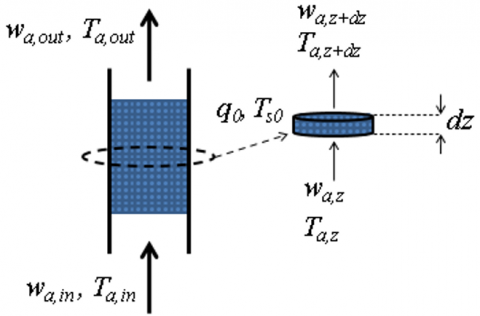Figure 1. Packed bed of adsorbent material

In this research, the laws of mass and energy conservation were applied to the thin silica gel vertical packed bed, given the following assumptions, to calculate the moisture and heat transfer:

1) The adsorbent material was silica gel of spherical shape and uniform in size.

2) The silica gel could adsorb only moisture from the process air.

3) The moisture concentration in the desiccant was constant along the r and θ axes but varied along the z axis.

4) The pressure drop of the thin silica gel packed bed was negligible.

5) The heat transfer through the packed bed was along the z-axis direction, and the column wall was of adiabatic wall.

6) The moisture dispersion along the z-axis direction was negligible and thus ignored.

A one-dimensional numerical model was developed to characterize the adsorption/desorption (dehumidification/ regeneration) moisture and heat transfer of silica gel vertical packed bed. Eqns. (1) and (2) are the mass and energy conservation equations on the desiccant side, and Eqns. (3) and (4) are the air-side mass and energy conservation equations, adapted from the work of Ramzy et al. . Our proposed model neglects mass and thermal diffusion inside the particles, since the reliable diffusivities are very rare [17, 18]. The variables’ definitions in these equations can be found in a nomenclature.

$\frac{\partial q}{\partial t}=\frac{{{{\dot{m}}}_{a}}}{{{m}_{s}}}({{w}_{a,z}}-{{w}_{a,z+dz}})$               (1)

${{k}_{b}}\frac{{{\partial }^{2}}{{T}_{s}}}{\partial {{z}^{2}}}+{{H}_{A}}{{h}_{m}}a\left( {{w}_{a}}-{{w}_{s}} \right)+ha\left( {{T}_{a}}-{{T}_{s}} \right)={{c}_{s}}{{\rho }_{s}}\left( 1-\varepsilon \right)\frac{\partial {{T}_{s}}}{\partial t}$                   (2)

$\frac{\partial {{w}_{a}}}{\partial t}=-\frac{{{{\dot{m}}}_{a}}}{{{\rho }_{a}}\varepsilon {{A}_{b}}}\frac{\partial {{w}_{a}}}{\partial z}-\frac{{{h}_{m}}a}{{{\rho }_{a}}\varepsilon }\left( {{w}_{a}}-{{w}_{s}} \right)$                 (3)

$\frac{\partial {{T}_{a}}}{\partial t}=-\frac{{{{\dot{m}}}_{a}}}{{{\rho }_{a}}\varepsilon {{A}_{b}}}\frac{\partial {{T}_{a}}}{\partial z}+\left( \frac{ha}{{{\rho }_{a}}{{c}_{a}}\varepsilon }+\frac{{{c}_{v}}{{h}_{m}}a}{{{\rho }_{a}}{{c}_{a}}\varepsilon }\left( {{w}_{a}}-{{w}_{s}} \right) \right)\left( {{T}_{s}}-{{T}_{a}} \right)$                  (4)

The numerical models (Eqns. (1)-(4)) are the differential equations, with the dehumidification and regeneration boundary and initial conditions as follows:

wa(z=0,t) = wa,in = wamb

Ta(z=0,t) = Tdeh,in (dehumidification)

Ta(z=0,t) = Treg,in (regeneration)

$\begin{matrix} {{\left. \frac{\partial {{T}_{s}}}{\partial z} \right|}_{z=0}}={{\left. \frac{\partial {{T}_{s}}}{\partial z} \right|}_{z=L}}=0 & {} \\\end{matrix}$

wa(z,t=0) = wa0

q(z,t=0) = q0

Ta(z,t=0) = Ta0

Ts(z,t=0) = Ts0

The simulated results in this research, dehumidification/ regeneration system was set up as a 10 cm-diameter and 15 cm-height cylindrical packed bed packed with 3.5 mm diameter spherical silica gel desiccants. The desiccant’s density was 1,200 kg·m-3 and the bed porosity (εb) was 0.35. The ambient air humidity (wamb) and temperature (Tamb) were 0.018 kgw·kga-1 and 30°C, respectively. The air inlet velocity (v) is 0.75 m·s-1. The equilibrium relative humidity was calculated from the work of Ramzy et al.  due to elevated regeneration temperatures (60, 70, 80, 90, 100, 110, 120°C). The auxiliary data are shown in the Table 1.

Table 1. Auxiliary data

 Auxiliary data HA=1,000(3,500-12,400q) q≤0.05 HA=1,000(2,950-1,400q) q≥0.05 cs=4,186q+921 ca=1,884wa+1,004(1-wa) cv=1,859+0.382Ta ks=0.37+0.97q+0.0014Ts  kb=kaεks1-ε, ka=0.029 W m-1 K-1 h=0.683ρavcaRe-0.51                   h=1.60ρavcaRe-0.42                         hm=0.70ρavRe-0.51                 hm=1.70ρavRe-0.42                 ${{w}_{s}}=\frac{0.622R{{H}_{s}}\text{ x }{{P}_{sat}}({{T}_{s}})}{{{P}_{a}}-R{{H}_{s}}\text{x }{{P}_{sat}}({{T}_{s}})}$ $\ln \,{{P}_{sat}}\left( kPa \right)=\,16.2886-\frac{3,816.44}{{{T}_{s}}\left( {}^\circ C \right)+227.02}$ RHs=55.61-2,069q+27,864q2-169,543q3+560,955q4     -968,999q5+682,782q6                RHs=0.78-5.759q+2,416q2-12,447q3+20,422q4           

The geometry of the adsorption/desorption scheme of this research is illustrated in Figure 2, consisting of one silica-gel vertical packed bed and two control valves. In adsorption, valve 1 was open and valve 2 remained shut (Figure 2a). On the other hand, in desorption, the valve status was reversed (Figure 2b).

## 2.png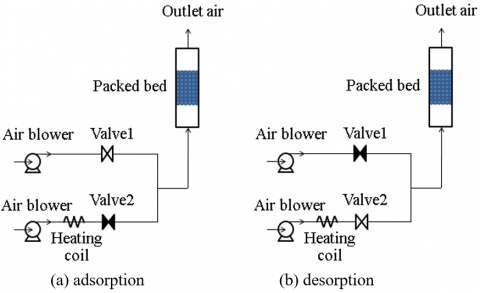Figure 2. The geometry of the adsorption/desorption scheme

2.2 Process performance

The performance of the silica gel packed bed was assessed by two parameters, namely the moisture removal capacity (MRC) and the dehumidification coefficient of performance (DCOP). MRC is the amount of water removal per cycle time (tcyc) and can be expressed as,

$MRC=\frac{{{{\dot{m}}}_{a}}}{{{t}_{cyc}}}{{\int\limits_{0}^{\Delta {{t}_{deh}}}{\left( {{w}_{a,in}}-{{w}_{a,out}} \right)}}_ {{}}}dt$         (5)

where, tcyc = ∆tdeh+∆treg.

DCOP is the ratio of latent heat during dehumidification to the sensible heat during regeneration, which can be expressed as:

$DCOP=\frac{LH\times {{{\dot{m}}}_{deh}}{{\int\limits_{0}^{\Delta {{t}_{deh}}}{\left( {{w}_{a,in}}-{{w}_{a,out\quad}} \right)\quad}}_{{}}}dt}{{{c}_{a}}\times {{{\dot{m}}}_{reg}}{{\int\limits_{0}^{\Delta {{t}_{reg}}}{\left( {{T}_{a,in,reg}}-{{T}_{a,amb\quad}} \right)\quad}}_{{}}}dt}$             (6)

where, LH is the latent heat of vapor in the process air, ∆tdeh and ∆treg are the cycle time of dehumidification and regeneration.

3. The Model Validation

Explicit finite difference was used to solve the nonlinear partial differential equations (Eqns. (1)-(4)). In the calculation, the thin silica-gel vertical packed bed was equally discretized into 100 layers, given that the grid mesh was less than 1.5 mm and 1 s time increment to ensure numerical stability and accuracy. The air humidity (wa), moisture content (q), air temperature (Ta), and silica gel temperature (Ts) of the grids were then calculated. Table 2 summarizes the experimental conditions using regular-density silica gel to verify the proposed numerical model.

Figure 3 illustrate the comparison between the calculated and experimental data of the exit air humidity (wa,out) of all four conditions during both absorption and desorption operations. While Figure 4 depicts the comparison between the calculated and experimental data of the exit temperature (Ta,out) during both absorption and desorption operations. The new model calculations are consistent with the experimental data and modestly better than the previous works [19, 21]. The detailed comparison can be found in our previous work .

Table 2. Data for verify the proposed numerical model

 Condition 1 2 3 4 Reference Ramzy  Pesaran  Ramzy  Pesaran  L (m) 0.055 0.0775 0.060 0.050 db (m) 0.16 0.13 0.16 0.13 ds (mm) 3.0 3.8 3.0 5.2 v (m s-1) 0.75 0.21 0.75 0.67 ε 0.35 0.31 0.35 0.31 ρs (kg m-3) 1,200 1,200 1,200 1,200 q0 (kgw kgs-1) 0.10 0.0417 0.283 0.26 Ts0 (⁰C) 31.0 23.3 34.5 25.4 wa,in (kgw kga-1) 0.020 0.010 0.018 0.0007 Tdeh,in (℃) 42.0 23.3 - - Treg,in (℃) - - 85.0 25.4

## 3.png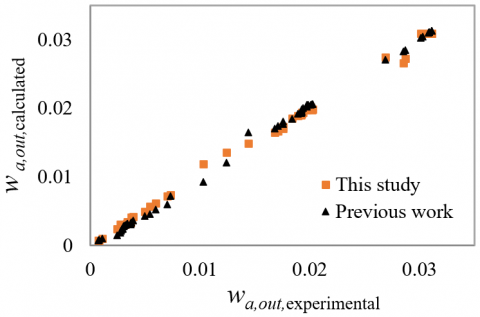Figure 3. Calculated and experimental data of wa,out

## 4.png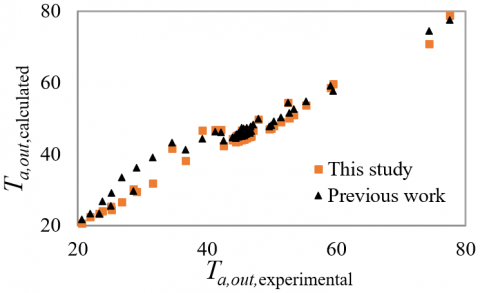Figure 4. Calculated and experimental data of Ta,out

4. Results and Discussion

Figure 5 illustrates the simulated wa,out, Ta,out and moisture transfer content (MTC) of the silica-gel packed bed during adsorption and desorption. The moisture transfer content (MTC) is the amount of moisture transfer between the air stream and the desiccant during adsorption and desorption at any given time. The moisture transfer content during adsorption (MTCad) and desorption (MTCde) are expressed as:

MTCde(t) = ρaAbv (wa,out(t)-wa,in(t)) for desorption             (8)

## 5.png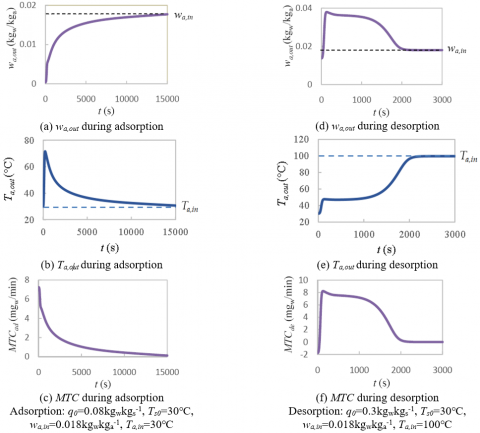Figure 5. Simulated wa,out, Ta,out and MTC of the silica-gel vertical packed bed during adsorption and desorption

In Figure 5a, wa,out was initially at the minimum and rose steadily prior to approaching the inlet air humidity (wa,in), where the silica gel was saturated. The elevated silica-gel temperature, as a result of heat of adsorption, caused the exit air temperature (Ta,out) to become warmer through convection heat transfer as shown in Figure 5b. The heat of adsorption decreased as the silica gel neared saturation. Subsequent inlet process air (30℃) gradually cooled down the bed temperature, causing Ta,out to fall. However, the elevated silica-gel temperature lowered the adsorption performance and MTCad. In Figure 5c, MTCadwas initially at the maximum due to low moisture content and low temperatures of the silica gel. It is observed that reaching saturation, wa,out and Ta,out approached wa,in and Ta,in, where MTCad proceeded to zero or there was no further adsorption.

At the start of desorption in Figure 5d, the silica gel temperature (Ts0) was lower than Ta,in (100℃), resulting in the lower vapor pressure on silica gel surface than that of the hot inlet air; and the subsequent air-to-desiccant moisture transfer. The air-to-desiccant moisture transfer (i.e., adsorption) caused the initial wa,out to be lower than wa,in. In Figure 5e, Ta,out was lower than Ta,in due to endothermic reactions. In addition, Ta,out was positively correlated to the desorption (regeneration) time. Initially, Ta,out steadily rose and the pace decelerated as it approached Ta,in. This was attributable to the initial higher rate of desorption. The initial negative MTC in Figure 5f, was due to adsorption, and reversed upon desorption as the silica gel temperature rose. The highest rate of desorption was the maximum MTCde, where wa,out was also highest. The rate of desorption and MTCde then declined until MTCde was zero as the vapor pressures on the silica gel surface and the hot inlet air were identical. Upon complete desorption, wa,out and Ta,out were identical to wa,in and Ta,in, respectively, and MTCde reached zero and no more water was desorbed.

Notably, both MTCad and MTCde were zero at each termination, in addition to Ta,out = Ta,in . These facts are later applied to develop TBC criteria to determine adsorption and desorption periods.

4.2 Conventional Steady Cyclic (CSC) operation

The Conventional Steady Cyclic (CSC) operation has long been the process of repetitive dehumidification and regeneration, where the cycle times of dehumidification (∆tdeh) and regeneration (∆treg) are usually set for simple operation. This limits its energy performance since it could easily fail to reach the break points.

Figure 6 illustrates the simulated wa,out and Ta,out of the CSC operation under variable regeneration temperatures (Treg): 60, 80, 100, and 120℃, and the operating cycle time (tcyc) of 180 min. The dehumidification/regeneration process proceeded in a successive fashion until the adsorbed and desorbed moistures were equal. In addition, the repetitive patterns of Ta,out and wa,out subsequently emerged as the dehumidification/ regeneration process advanced.

## 6a.png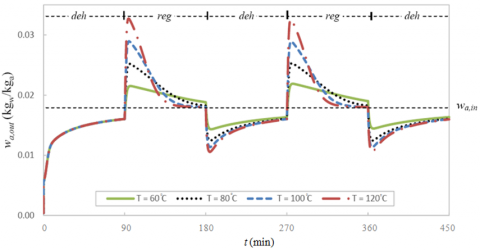(a) wa,out of the CSC operation

## 6b.png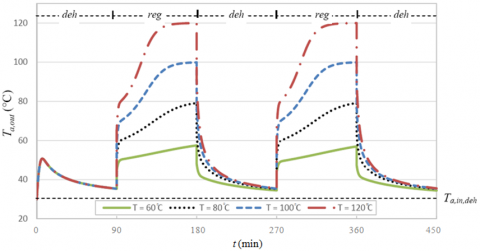(b) Ta,out of the CSC operation

Figure 6. The simulated wa,out and Ta,out of the CSC operation under variable regeneration temperatures (Treg)

In Figures 6a-b, wa,out was initially, substantially low due to low silica gel temperatures and moisture content, rendering it highly effective in dehumidification. In regeneration, as the thermal air was fed into the silica-gel packed bed, wa,out and Ta,out rose. Both wa,out and Ta,out declined in subsequent dehumidification. Nevertheless, the dehumidification efficiency was lower due to high silica gel temperatures from the previous regeneration.

Specifically, in Figure 6b, at Treg of 100 and 120℃, Ta,out during the first regeneration (90-180 min) was equal to Ta,in well before the end of cycle time (180 min). At 60℃, Ta,out was lower than Ta,in, hindering the moisture removal from the silica gel. The optimal Treg was thus 80°C since Ta,out and Ta,in were identical at the end of cycle time, indicating that the thermal inlet air was most efficiently utilized in the silica gel regeneration.

In fact, ambient air humidity (wamb) and temperatures (Tamb) vary with times of day, giving rise to variation in the optimal Treg and tcyc. Also, the simple CSC technique suffered from high energy costs. Thus, the subsequent section discusses an alternative energy-efficient regeneration strategy to remove moisture from silica gel.

4.3 Temperature Breakpoint Cyclic (TBC) operation

4.3.1 Temperature breakpoint

Temperature swing adsorption (TSA) is a process that utilizes thermodynamics principle in adsorption and desorption. Specifically, adsorption is more effective at lower temperatures whereas desorption is more effective at higher temperatures. In general, the adsorption performance of packed beds is determined by breakthrough curve. In adsorption, the adsorbent never reaches the saturation point to prevent contaminating of the adsorbate into the exit gas. Upon arriving at the breakpoint, the operating mode was switched from adsorption to desorption. The breakpoint is defined as the time that the adsorbate is allowed to be released from the adsorbent into the gas at an acceptable concentration.

In TSA dehumidification, it is nevertheless less efficient to rely on air humidity to alternate between the dehumidification and regeneration modes, given that the air humidity measured by a hygrometer is relative humidity (%) and temperature (℃), not absolute humidity (wa) in unit of kgw/kga. As a result, simple and accurately measured temperature breakpoint is a more practical alternative for the mode alteration.

In Figures 5 and 6, during adsorption/desorption (dehumidification/regeneration), Ta,out varied in direct proportion to absolute exit air humidity (wa,out). If the relation could be established, the breakpoint could thus be determined by temperature breakpoint (Tb) in lieu of absolute-humidity breakpoint. The dimensionless differences of absolute air humidity in adsorption (w*ad); and air temperatures in adsorption (T*ad) and desorption (T*de) are, respectively, expressed in Eqns. (9), (10), and (11). They represent the degree of difference between the inlet and outlet interest compared to the inlet one.

${{w}^{*}}_{ad}=\frac{{{w}_{a,in}}-{{w}_{a,out}}}{{{w}_{a,in}}}$ for adsorption            (9)

${{T}^{*}}_{ad}=\frac{{{T}_{a,out}}-{{T}_{a,in}}}{{{T}_{a,in}}}$ for adsorption             (10)

${{T}^{*}}_{de}=\frac{{{T}_{a,in}}-{{T}_{a,out}}}{{{T}_{a,in}}}$ for desorption            (11)

From Eqns. (10)-(11), the adsorption and desorption temperature breakpoints (Tbad and Tbde), Ta,out when wa,out is an acceptable concentration, could be calculated by:

$T_{ad}^{b}={{T}_{a,in}}\left( 1+{{T}^{*}}_{ad} \right)$ for adsorption                (12)

$T_{de}^{b}={{T}_{a,in}}\left( 1-{{T}^{*}}_{de} \right)$ for desorption          (13)

Figures 7 and 8 respectively illustrate the simulated w*ad relative to T*ad in adsorption; and MTCde relative to T*de in desorption, under variable parametric conditions. The base case was characterized by wa,in of 0.018kgwkga-1, Tdeh,in of 30℃, Treg,in of 100℃, air flow velocity (v) of 0.75ms-1, initial moisture content (q0) of 0.08kgwkgs-1, silica-gel packed bed length (L) of 15cm, and silica gel diameter (ds) of 3.5mm. At low T*adand T*de (below 0.1), w*ad and MTCde minimally varied in response to the parametric variation. Nonetheless, the responsiveness was more pronounced with increase in T*ad and T*de. Notably, the temperature breakpoint (Tb) could be approximated by the slope.

Specifically, in adsorption, the absolute-humidity breakpoint was the dimensionless difference of absolute air humidity (w*ad) at any given dimensionless difference of temperature (T*ad) (Figure 7). Meanwhile, in desorption, the silica gel performance was assessed by the moisture transfer content (MTCde) at any given dimensionless difference of temperature (T*de) (Figure 8). The lower the MTCde, the lower the moisture content in silica gel and subsequent higher adsorption efficiency. Thus, the effective desorption temperature breakpoint (Tbde) lies in the low T*de range.

4.3.2 Temperature Breakpoint Cyclic (TBC) operation

In Figure 6b, at Treg of 100 and 120°C, in regeneration, the temperature-specific complete desorption occurred where Ta,out and Ta,in were identical. In dehumidification, the adsorption was deliberately terminated prior to full capacity, as evidenced by higher Ta,out in relation to Ta,in. Thus, the performance of the silica-gel packed bed could be enhanced by manipulating the adsorption and desorption temperature breakpoints (Tbad and Tbde) to lengthen the adsorption time, shorten the desorption time, or both.

## 7.png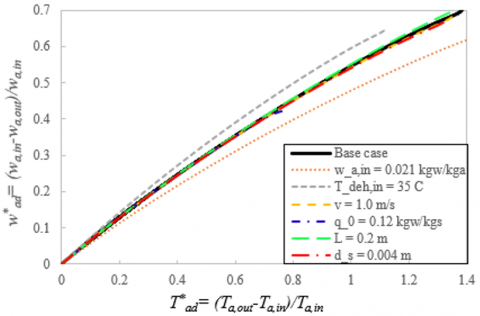## 8.png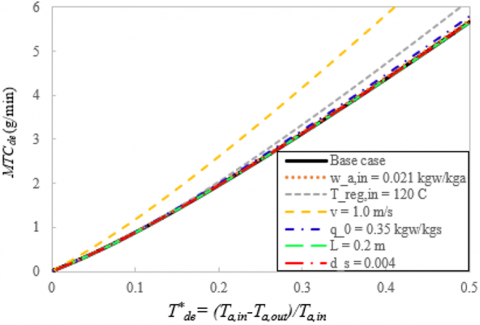Figure 8. Simulated MTCde relative to T*de in desorption under variable parametric conditions

## 9a.png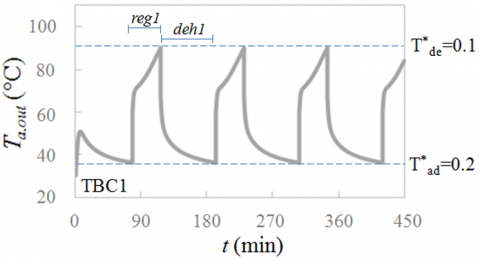(a) TBC1: T*ad = 0.2, T*de= 0.1

## 9b.png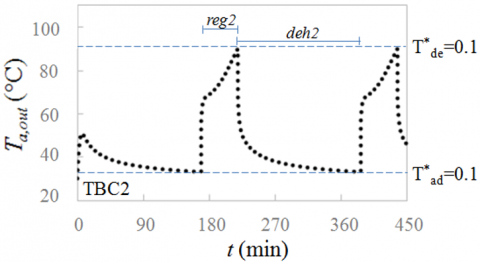(b) TBC2: T*ad = 0.1, T*de= 0.1

## 9c.png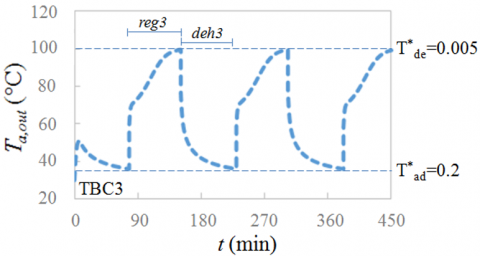(c) TBC3: T*ad = 0.2, T*de= 0.005

## 9d.png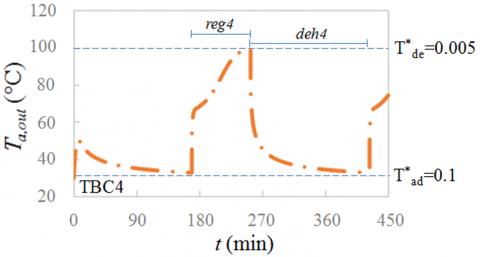(d) TBC4: T*ad = 0.1, T*de= 0.005

Figure 9. The simulated TBC operation at Treg of 100°C

The TBC operation is the process whereby Tbad and Tbdeare manipulated to regulate the control valves that alternate between the dehumidification and regeneration modes. Figure 9 illustrates the simulated TBC operation for 450 min at Treg of 100°C, given T*ad and T*dewere varied between 0.1 and 0.2; and 0.005 and 0.1, respectively. The TBC profile (Figure 9) resembled that of the CSC (Figure 6).

At T*ad of 0.2 (Figures 9a, c), the dehumidification time was shorter than under T*ad = 0.1 (Figures 9b, d). In regeneration, at T*de of 0.1 (Figures 9a, b), the regeneration time was also shorter than under T*de is 0.005 (Figures 9c, d). In Figure 9, the dehumidification and regeneration durations (dehiand regi) were subject to variations in T*ad and T*de, regardless of variable ambient air humidity (wamb) and temperatures (Tamb).

4.4 Performance of silica gel packed bed

The performance of the silica-gel packed bed was determined by the moisture removal capacity (MRC) and dehumidification coefficient of performance (DCOP). Table 3 tabulates the simulation conditions under the Conventional Steady Cyclic (CSC) and Temperature Breakpoint Cyclic (TBC) operations.

Table 3. The simulation conditions

 Operation Condition CSC1 tcyc= 90min CSC2 tcyc= 180 min TBC1 T*ad = 0.2, T*de=0.1 TBC2 T*ad = 0.1, T*de=0.1 TBC3 T*ad = 0.2, T*de=0.005 TBC4 T*ad = 0.1, T*de=0.005

Figure 10 illustrates the MRC and DCOP of the packed bed under variable regeneration temperatures (Treg): 60, 70, 80, 90, 100, 110, and 120°C. In Figure 10a, the MRC was positively correlated to Treg since silica gel desorbed more efficiently at higher Treg. Specifically, the MRC of CSC1 was higher than that of CSC2, due to the former’s shorter cycle time (90 vs 180 min).

At T*ad of 0.1 (TBC2 and TBC4), the overall MRC of TBC2 and TBC4 were comparably low. This was attributable to the silica gel approaching saturation toward the end of adsorption and thus the lower adsorption rate. On the other hand, at T*ad of 0.2 (TBC1 and TBC3), their overall MRC improved. The highest MRC was achieved under TBC1 due to the comparably high T*ad (0.2) and high T*de (0.1), which in turn accelerated the dehumidification and regeneration. In the figure, under TBC3 and TBC4 (T*de = 0.005), at low Treg (60, 70, 80°C) the MRC were very low due to the extended regeneration time as a result of the low T*de.

As evident in Figure 10a, the MRC alone is inadequate for judging the merit of TBC over CSC because all of the simulation conditions (CSC1-2 & TBC1-4) achieved comparable MRC, especially under CSC1 and TBC1 where their MRC were almost identical. Another performance indicator (i.e., DCOP) was thus proposed.

In Figure 10b, under CSC, the DCOP was inversely correlated to Treg. This was due to high energy consumption required to heat the air temperature during regeneration. On the other hand, under TBC, DCOP gradually rose with increase in Treg. This was attributable to the shorter regeneration time as Treg increased. Under TBC3 and TBC4, where T*de was low (0.005), the DCOP were lower than that of TBC1 and TBC2, where T*de was high (0.1). Specifically, at the lower T*de, the regeneration duration was substantially longer, and thus the higher energy consumption. By comparison, TBC outperformed CSC regarding more energy efficiency, as evidenced by the former’s larger DCOP.

Notably, at the maximum Treg of 120°C, the MRC and DCOP associated with the TBC operation were approximately 10 and 100% higher than CSC’s. The findings suggested that both operations could achieve comparable MRC while the energy cost could be slashed by half under TBC, as evidenced by the DCOP.

## 10a.png(a) moisture removal capacity (MRC)

## 10b.png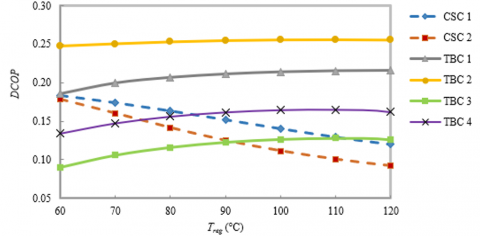(b) dehumidification coefficient of performance (DCOP)

Figure 10. Simulated performance of silica gel packed bed

5. Conclusions

This research proposed a novel desiccant packed-bed dehumidification system based on the Temperature Breakpoint Cyclic (TBC) operation. In the study, a one-dimensional unsteady model of the cyclic dehumidification system has been developed and validated. The performance of the Conventional Steady Cyclic (CSC) operations have then been analyzed under various cycle times and regeneration temperatures. A weakness of the CSC operation has been pointed out. A Temperature Breakpoint Cyclic (TBC) operation was then proposed and evaluated. By comparison, the TBC scheme outperformed the CSC with regard to the dehumidification capability and energy efficiency, as evident by the higher MRC and DCOP. Moreover, under CSC, the cycle time needs to be optimized based on an unrealistic assumption of constant ambient air humidity (wamb) and temperature (Tamb). In fact, wamb and Tamb vary with times of day. Furthermore, silica gel deteriorates with use, decreasing the accuracy of the optimized cycle time. Meanwhile, under TBC, the dehumidification and regeneration modes could be dynamically adjusted based on the different air temperatures (T*). The results can also be applied to the other absorption/desorption operations by modifying the model auxiliary data according to each system. This research nevertheless falls short of optimization of T*. As such, subsequent research would investigate the optimal T*ad and T*de of the silica gel-based TBC operation as well as the application of the TBC operation on the other commercially available adsorption processes.

Acknowledgment

The authors would like to express sincere gratitude to Asst. Prof. Dr. Amata Anantpinijwatna for his solid and invaluable comments and the School of Engineering, King Mongkut’s Institute of Technology Ladkrabang (KMITL), Thailand, for the contributions to this research.

Nomenclature
 a volumetric surface area, m-1 Ab bed cross section area, m2 c specific heat, J kg-1 K-1 d diameter, m DCOP dehumidification coefficient of performance h convective heat transfer coefficient, W m-2 K-1 hm convective mass transfer coefficient, kg m-2 s-1 HA heat of adsorption, J kg-1 k thermal conductivity, W m-1 K-1 L bed length, m LH latent heat of vapor, J kg-1 m mass, kg $\dot{m}$ mass flow rate, kg s-1 MRC moisture removal capacity, gw min-1 MTC moisture transfer content, gw min-1 P Pressure, kPa Psat saturated water vapor pressure, kPa q moisture content, kgwkgs-1 R particle radius, m Re Renolds number, Re = 2Rv$\nu$-1 RHs relative air humidity, % t time, min T temperature, °C Tb temperature breakpoint, °C T* dimensionless difference of air temperature v air flow velocity, m s-1 wa air humidity, kgwkga-1 ws equilibrium air humidity, kgwkga-1 wa* dimensionless difference of absolute air humidity z axial position, m ∆t period, min Greek symbols $\rho$ density, kg m-3 $\varepsilon$ bed porosity $\nu$ kinematic viscosity, m2 s-1 Subscripts 0 initial condition a air ad adsorption amb ambient b packed bed de desorption deh dehumidification in inlet out outlet p particle reg regeneration s silica gel v water vapor w water z axial direction
References

 Genco, A., Viggiano, A., Magi, V. (2018). How to enhance the energy efficiency of HVAC systems. Mathematical Modelling of Engineering Problems, 5(3): 153-160. https://doi.org/10.18280/mmep.050304

 Rambhad, K.S., Walke, P.V., Tidke, D.J. (2016). Solid desiccant dehumidification and regeneration methods-A review. Renewable and Sustainable Energy Reviews, 59: 73-83. https://doi.org/10.1016/j.rser.2015.12.264

 Chebbi, R., Qasim, M., Jabbar, N.A. (2019). Optimization of triethylene glycol dehydration of natural gas. Energy Reports, 5: 723-732. https://doi.org/10.1016/j.egyr.2019.06.014

 Misha, S., Mat, S., Ruslan, M.H., Sopian, K. (2012). Review of solid/liquid desiccant in the drying applications and its regeneration methods. Renewable and Sustainable Energy Reviews, 16(7): 4686-4707. http://dx.doi.org/10.1016/j.rser.2012.04.041

 Zouaoui, A., Ghedira, L.Z., Nasrallah, S.B. (2016). Experimental investigation of air dehumidification and regeneration operations using packed bed of silica gel particles. International Journal of Heat and Technology, 34(1): 103-109. https://doi.org/10.18280/ijht.340115

 Alami, A., Boucham, B., Gouareh, A. (2019). Investigation on the energy efficiency of a geo-sol adsorption heat transformer in the Algerian context. International Journal of Heat and Technology, 37(3): 820-830. https://doi.org/10.18280/ijht.370319

 Sawardsuk, P., Jongyingcharoen, J.S., Cheevitsopon, E. (2020). Evaluation of cyclic efficiency of multilayer desiccant bed column. E3S Web of Conferences, 187: 04018. https://doi.org/10.1051/e3sconf /202018704018

 Rogala, Z., Kolasiński, P., Błasiak, P. (2018). The influence of operating parameters on adsorption/desorption characteristics and performance of the fluidised desiccant cooler. Energies, 11(6): 1597. https://doi.org/10.3390/en11061597

 Wu, X.N., Ge, T.S., Dai, Y.J., Wang, R.Z. (2018). Review on substrate of solid desiccant dehumidification system. Renewable and Sustainable Energy Reviews, 82: 3236-3249. https://doi.org/10.1016/j.rser.2017.10.021

 Shamim, J.A., Hsu, W.L., Kitaoka, K., Paul, S., Daiguji, H. (2018). Design and performance evaluation of a multilayer fixed-bed binder-free desiccant dehumidifier for hybrid air-conditioning systems: Part I–experimental. International Journal of Heat and Mass Transfer, 116: 1361-1369. https://doi.org/10.1016/j.ijheatmasstransfer.2017.09.051

 Berg, F., Pasel, C., Eckardt, T., Bathen, D. (2019). Temperature swing adsorption in natural gas processing: A concise overview. ChemBioEng Reviews, 6(3): 59-71. https://doi.org/10.1002/cben.201900005

 Petryka, M.R., Khimich, A., Petryk, M.M., Fraissard, J. (2019). Experimental and computer simulation studies of dehydration on microporous adsorbent of natural gas used as motor fuel. Fuel, 239: 1324-1330. https://doi.org/10.1016/j.fuel.2018.10.134

 Mesfer, M.K.A., Danish, M., Khan, M.I., Ali, I.H., Hasan, M., Jery, A.E. (2020). Continuous fixed bed CO2 adsorption: breakthrough, column efficiency, mass transfer zone. Processes, 8: 2133. https://doi.org/10.3390/pr8101233

 Kannan, V.S., Arjunan, T.V., Vijayan, S. (2020). Experimental investigation of temperature swing adsorption system for air dehumidification. Heat and Mass Transfer, 56: 2093-2105. https://doi.org/10.1007/s00231-020-02841-w

 Nastaj, J., Ambrozek, B. (2012). Modeling of drying of gaseous mixtures in TSA system with fixed bed of solid desiccants. Drying Technology, 30: 1062-1071. https://doi.org/10.1080/07373937.2012.685138

 Ramzy, A.K., Babu, T.P.A., Kadoli, R. (2011). Semi-analytical method for heat and moisture transfer in packed bed of silica gel. International Journal of Heat and Mass Transfer, 54(4): 983-993. https://doi:10.1016/j.ijheatmasstransfer.2010.09.007

 Gurgel, J.M., Filho, L.S.A., Grenier, P., Meunier, F. (2001). Thermal diffusivity and adsorption kinetics of silica-gel/water. Adsorption, 7: 211-219. https://doi.org/10.1023/A:1012732817374

 Mamaliga, I., Schabel, W., Petrescu, S. (2010). Characterization of water vapour diffusion into spherical silica gel particles. Revista De Chimie, 61(12): 1231-1234.

 Ramzy, A.K., Kadoli, R., T.P., A.B. (2013). Experimental and theoretical investigations on the cyclic operation of TSA cycle for air dehumidification using packed beds of silica gel particles. Energy, 56: 8-24. https://doi.org/10.1016/j.energy.2013.03.048

 Pesaran, A.A., Mills, F. (1987). Moisture transport in silica gel packed beds-I Theoretical study. International Journal of Heat and Mass Transfer, 30(6): 1037-1049. https://doi.org/10.1016/0017-9310(87)90034-2

 Pesaran, A.A., Mills, F. (1987). Moisture transport in silica gel packed beds-II Experimental study. International Journal of Heat and Mass Transfer, 30(6): 1051-1060. https://doi.org/10.1016/0017-9310(87)90035-4

 Murathathunyaluk, S., Junjiewchai, J., Kitchaiya, P. (2019). Effect of regeneration conditions on dehumidification desiccant packed bed. Chemical Engineering Transactions, 76: 715-720. https://doi.org/10.3303/CET1976120